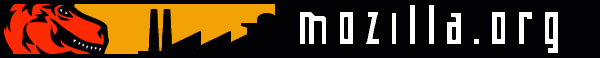]> MathML in ActionHome | Projects | Docs | Jargon Bugzilla | LXR | Tree Status | Checkins Feedback | FAQ | Search

## MathML in Action[ Languages: English - Hebrew - Thai ]

You already have a MathML-enabled build but what you see on the screenshot is not what you get? In that case you are probably missing some crucial MathML fonts.

Now that you are well-equipped, you should be able to see this inline equation with varying accents: $x ^ + xy ^ + xyz ^ .$ Next to it is this tiny formula, $det | a c b d | = a d - b c ,$ which can also be typeset in displaystyle as $det | a b c d | = a d - b c .$

Mathematical typesetting is picky. MathML in Mozilla aims at complying with the MathML specification so that What You See Is What You Markup, or to put it another way What You See Is What You Made, or in short "WYSIWYM". The difference between these two is in the markup! $( ... ( ( a 0 + a 1 ) n 1 + a 2 ) n 2 + ... + a p ) n p$ $( ... ( ( a 0 + a 1 ) n 1 + a 2 ) n 2 + ... + a p ) n p$

The roots of this bold equation $y 3 + p y + q = 0$ are also bold $y = - q 2 + q 2 4 + p 3 27 2 3 + - q 2 - q 2 4 + p 3 27 2 3 .$

As for the roots of the equation $a x 2 + b x + c = 0 ,$ click anywhere in the yellow area to zoom-in/zoom-out using RESTYLE: $x = - b ± b 2 - 4 a c 2 a$

Consider an interesting markup like this or other complex markups like these $Ell ^ Y ( Z ; z , τ ) := ∫ Y ( ∏ l ( y l 2 π i ) θ ( y l 2 π i - z ) θ ′ ( 0 ) θ ( - z ) θ ( y l 2 π i ) ) × ( ∏ k θ ( e k 2 π i - ( α k + 1 ) z ) θ ( - z ) θ ( e k 2 π i - z ) θ ( - ( α k + 1 ) z ) )$ $π ( n ) = ∑ m = 2 n ⌊ ( ∑ k = 1 m - 1 ⌊ ( m / k ) / ⌈ m / k ⌉ ⌋ ) - 1 ⌋$ $∥ ϕ ∥ W s k ( Ω g ) ≝ ( ∑ | α | ≦ k ∂ α ϕ ∂ ξ α L s ( Ω g ) s ) 1 / s$

For more examples, refer to links on the MathML Project page, and if you are building your own Mozilla binary, see the directory mozilla/layout/mathml/tests.

Now, what next? As you try out MathML in Mozilla, what to do with those few things that appear to you not to work as per the MathML spec? Or what about those things that just seem itchy, and that you wish could be done just a little better? Or what about those things that were working before and are not working anymore (a.k.a. regressions)? In either case, head over to Bugzilla to report the discrepancies. Bugzilla has a big memory for these things, and besides, how would your problems be fixed if they are not reported?!

Getting involved is part of your contribution towards enriching Gecko with an elegant standards-compliant MathML renderer. Your feedback can be manifested by putting MathML content on the web, reporting bugs in Bugzilla, and, if you can help with code, inspecting/improving the current code, and/or picking up an item in the ToDo list.

This page is typeset according to XHTML, the reformulated way to go for the web.